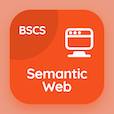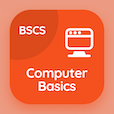Computer Science Courses Online

Database Systems Prep Tests

Database Systems Tests

The e-Book UML Diagram Multiple Choice Questions (MCQ Quiz), UML Diagram quiz answers PDF to learn online courses, database systems tests. Study Database Design and ER Model Multiple Choice Questions and Answers (MCQs), UML Diagram quiz questions for accelerated computer science degree online. The e-Book "UML Diagram MCQ" App Download: constraints in dbms, entity relationship diagrams, specialization test prep for computer and information science.

The MCQ "The UML diagram that specifies the sequences/ steps of operations to be performed" PDF, UML Diagram App Download (Free) with class diagram, use case diagram, activity diagram, and e-r diagram choices for accelerated computer science degree online. Practice uml diagram quiz questions, download Google eBook (Free Sample) for bachelor's degree in computer science.

## Database Systems: UML Diagram MCQs

MCQ: The UML diagram that specifies the sequences/ steps of operations to be performed

A) Class diagram
B) Use case diagram
C) Activity diagram
D) E-R diagram

MCQ: The UML diagram that depicts the hardware system component level and its interconnections, is said to be

A) E-R diagram
B) Implementation diagram
C) Activity diagram
D) Use case diagram

MCQ: Some basic UML have defined parts of types

A) 2 types
B) 3 types
C) 4 types
D) 5 types

MCQ: The UML diagram that shows the interaction between users and the system, is known as

A) Activity diagram
B) E-R diagram
C) Use case diagram
D) Class diagram

MCQ: The UML diagram that depicts the software system component level and its interconnections, is said to be

A) Implementation diagram
B) Activity diagram
C) Use case diagram
D) Class diagram

### Practice Tests: Database Systems Exam Prep

Download Database Management System Quiz App, Semantic Web MCQs App and Computer Basics MCQ App for Android & iOS devices. These Apps include complete analytics of real time attempts with interactive assessments. Download Play Store & App Store Apps & Enjoy 100% functionality with subscriptions!